##1. Generalized isogonal transformations, definition

Quadratic transformations are related to families of conics or complete quadrilaterals. Later generate the family of conics passing through their vertices. Below I consider the case of conics passing through four points in general position for which the two aspects are equivalent.
Given a family of conics, and a point P, all polars of P with respect to the conic-members of the family pass through another point P' = F(P).
This defines the quadratic transformation (or generalized isogonal transformation or generalized conjugacy) of the family. In practice, P' is constructed as the intersection point of two polars L1, L2 of P with respect to two fixed member-conics of the family. In the definition below I use two degenerate members of such a family.

A particular case of quadratic transformations are the generalized isogonal transformations P' = tK(P) with respect to a triangle ABC and a point K on its plane and not lying on its side-lines. These are defined geometrically as follows:
 Construct the harmonic associates {A',B',C'} of K (see Trilinears.html ).
 From the bundle of conics passing through {K,A',B',C'} single out the two degenerate conic-members c1(KA',B'C') and c2(KB',C'A').
 To each point P, associate the intersection point P' of the polars of P with respect to c1 and c2.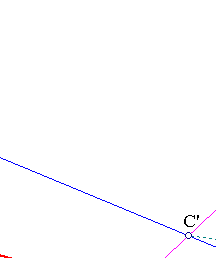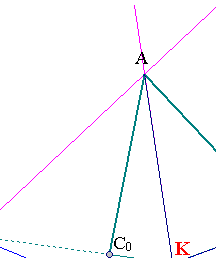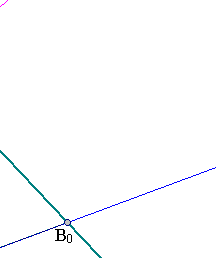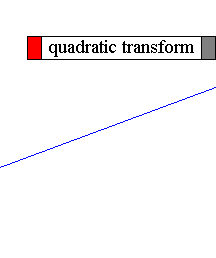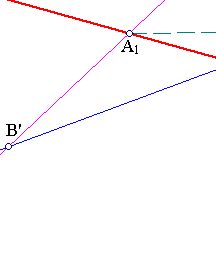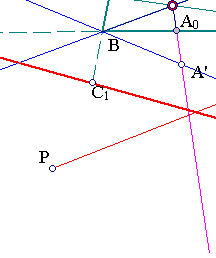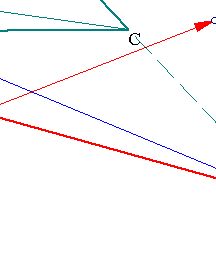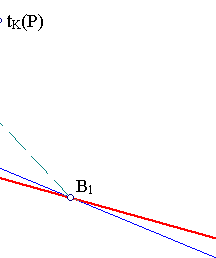Taking the system of points {A,B,C,K} as a projective base, the generalized isogonal transformation tK is easily calculated in the respective projective coordinates to have the description:
tK(x,y,z) = (1/x, 1/y, 1/z).
Thus K and its harmonic associates {(-1,1,1),(1,-1,1),(1,1,-1)} are the only fixed points of tK.
The tripolar of K is also easily seen to have the equation:
x+y+z=0.

##2. Tripolars of general points

For another point referred to the same coordinate system P(a,b,c) the corresponding tripolar is easily calculated to have the equation :
(1/a)x + (1/b)y + (1/c)z = 0. Thus in every such system the operation of taking the trilinear polar of a point P(a,b,c) has the same expression in coordinates: [(a,b,c) ---> line with coefficients (1/a,1/b,1/c)]. This shows also that the operation is not a projective map (or correlation as is usually named a projectivity between the space of points and the space of lines).

##3. Lines transform to conics

The quadratic transform tK applied to a line
L : ax+by+cz=0
transforms it to a conic passing through the vertices of the triangle.
In fact, setting x' = 1/x, y' = 1/y, z' = 1/z and considering the image of the line (L: ax+by+cz=0) under the transformation, we see that this satisfies
a/x' + b/y' + c/z' = 0 <==> ay'z' + bx'z' + cx'y' = 0,
which is the equation of a conic passing through the vertices of the triangle.
In particular, the trilinear polar of K having equation x+y+z=0 transforms to the conic
(c) : (1/x)+(1/y)+(1/z) = yz+zx+xy=0.
The first form of the equation shows also that a point P(x,y,z) on (c) has a trilinear polar passing through K:
(1/x)+(1/y)+(1/z) = 0 <==> line (1/x)u+(1/y)v+(1/z)w=0 passes through (u,v,w)=(1,1,1).
Another way to say it (see below) is that: conic (1/x)+(1/y)+(1/z)=0 is generated by the tripoles of lines through K.

##4. Various interpretations

The symmetry of the previous relations allows for various interpretations:
 The (generalized) isogonals tK(P) of points P on a line ax+by+cz=0 are on the conic ayz+bxz+cxy=0.
 The trilinear polar tr(P) of a point P(x,y,z) on the conic a/x+b/y+c/z=0 is a line with coefficients (1/x,1/y,1/z), hence passes through point (a,b,c). Equivalently:
 The conic consists of the tripoles of all lines through point (a,b,c), which is the isogonal conjugate of (1/a,1/b,1/c) which has ax+by+cz=0 as trilinear polar (see IsotomicConicOfLine.html for a figure illustrating the case).

##5. An induced transformation

Connected with the generalized isogonal transformation F=tK, defined in (1), is a transformation for lines using the intercepts with the sides of the basic triangle. The setting for this transformation is the following:
 Let ABC be a triangle and {K,LK} a point not on the side-lines of the triangle and its trilinear polar LK.
 Consider also the traces {A0,B0,C0} of the cevians through K on sides respectively {BC,CA,AB} as well as their harmonic conjugates {A1,B1,C1} (which are points on LK) with respect to pairs respectively {(B,C),(C,A),(A,B)}.
 For each line L intersecting the sides of the triangle at points {A',B',C'} respectively lying on {BC,CA,AB} find their harmonic conjugates {A'',B'',C''} with respect to pairs {(A0,A1),(B0,B1),(C0,C1)}.
 {A'',B'',C''} are on a line L'. If {A',B',C'} are created by line ax+by+cz=0, then {A'',B'',C''} are created by line (1/a)x+(1/b)y+(1/c)z=0. In other words the tripolar L = tr(P) of the point P(1/a,1/b,1/c) which is ax+by+cz=0, maps by this transform to the tripolar L'=F*(L)=F*(tr(P)) = tr(F(P)) of the isogonal conjugate Q(a,b,c) of P, which is (1/a)x+(1/b)y+(1/c)z=0.
 The transformation on lines L'=F*(L) thus defined makes sense for every line not passing through the vertices of ABC. Besides it is involutive and together with the isogonal transformation and the trilinear polar construction L=tr(P) which to P(a,b,c) associates its trilinear polar (1/a)x+(1/b)y+(1/c)z=0 makes the following diagram of transformations commutative.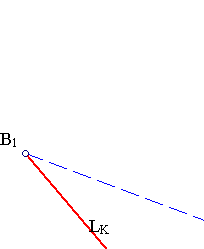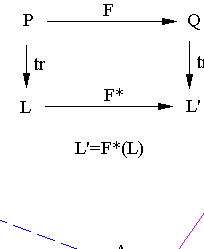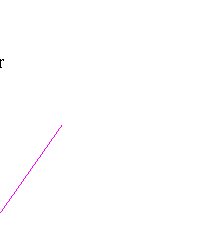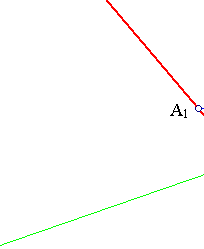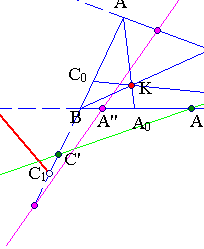Remark Using the F* one can walk the diagram around and define F using trilinear polars through F(P) = tr-1(F*(tr(P))). This is not essentially different from the initial definition since both use appropriate operations of taking harmonic conjugate points with respect to pairs of points. It gives though another interesting aspect of the transformation F.

##6. The line at infinity

The induced transformation F* can be used to prove that the middles {A2,B2,C2} of the segments correspondingly {A0A1,B0B1,C0C1} are on a line L. In fact by definition this line must be the image via F* of the line at infinity, which, invariantly for every triangle, is the tripolar of the centroid G (thus having the equation (1/g1)x+(1/g2)x+(1/g3)x=0, G=(g1,g2,g3) being the coordinates of G in the generalized system). This is a trivial (but exotic) proof of Newton's theorem (see Newton.html ) on the middles of diagonals of a complete quadrilateral (it is easy to locate the appropriate quadrilateral in the figure below). As a bonus one can find the conjugate F(G) = tr(L), as the tripole of L.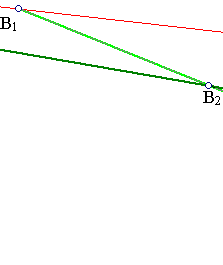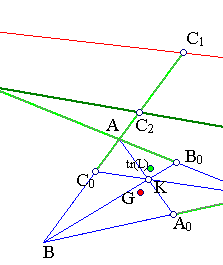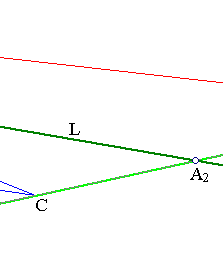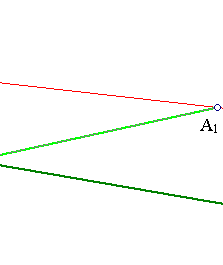##7. The dual picture

Denote by P0* the bundle of lines through P0 and by ~P the dual line of P defined as the tr(F(P)).
F* induces a projective map between the two projective lines: P0* and ~P0:
An element of P0* i.e. a line L: ax+by+cz = 0 , passing through point P0(x0,y0,z0), thus satisfying
ax0+by0+cz0=0,
maps via F* to line L': (1/a)x+(1/b)y+(1/c)z=0 which is the trilinear polar of point Q(a,b,c).
Thus, the tripole Q of L'=F*(L) is on line x0x+y0y+z0z=0, which is the tripolar of (1/x0,1/y0,1/z0), i.e. Q is an element of ~P0.
In (4) we had also a line L turning around a point P0 (i.e. passing through it) and saw that the tripoles generate a conic (c). Next section investigates the dual phenomenon. A point Q glides on a line and we show that the corresponding trilinear polars LQ envelope a conic related to (c), namely the conic tangent to the sides of the triangle and passing through {A0,B0,C0}. There is a geometric proof of this, whose discussion starts in IncircleTangents.html , but I give below another proof based on coordinates.

##8. The tangents of inconics

To complement the previous list of aspects of the generalized isogonal transformation in combination with the trilinear-polars we have to find out what are the tripolars of points lying on a line ax+by+cz=0.
To simplify matters assume the figure of (1) with coordinate basis {A,B,C,K} and line x+y+z=0 the trilinear polar of K. Then parametrize this line through two points, for example A-B and A-C. Every other point of LK is represented as
(A-B) -t(A-C) = (1-t)A + (-1)B + (t)C,
which has corresponding trilinear polar
(1/(1-t))x + (-1)y + (1/t)z =0, which is equivalent to (t)x + (-t(1-t))y + (1-t)z=0.
Eliminate t to find the envelope of these lines by taking the derivative:
x + (2t-1)y - z =0 => t = (y+z-x)/(2y).
This, introduced to the previous equation and simplifying reduces it to
-x2 - y2 -z2 + 2yz + 2zx + 2xy = 0.
It is easy to see that this conic passes through {A0=B+C, B0=C+A, C0=A+B} and has there tangents the coordinate axes respectively {x=0, y=0, z=0}.
See the file InconicsTangents.html for references to geometric proofs of the same property.
Note that the matrices representing the conics as quadratic forms are respectively: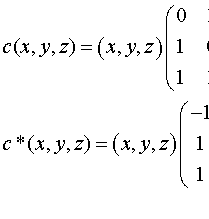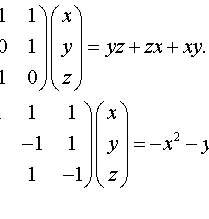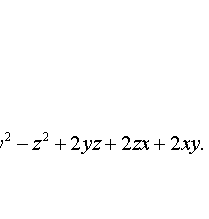The matrices have a product which is a multiple of the identity matrix (by the factor 2). Thus the conics they represent are dual to each other (see TriangleConics.html for a related discussion).

##9. Projective invariance

Consider two systems {A1B1C1, K1} and {A2B2C2, K2} each composed by a triangle and a point not lying on the side-lines of that triangle. For every two such systems there is a unique projectivity (F) mapping the one system onto the other i.e. mapping the vertices of the triangle A1B1C1 to corresponding vertices of the triangle A2B2C2 and also mapping K1 to K2.
All the constructions made in order to define the quadratic transformation or conjugacy are preserved by projective transformations. Thus denoting by {k1, k2} the corresponding conjugacies we obtain the commutative diagram: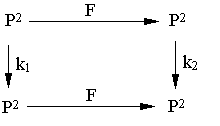P2 denotes here the projective plane. Since taking the trilinear polar with respect to a triangle is also preserved by projectivities, we get the commutative diagram tr2*F = F*tr1, where {tr1, tr2} denotes the operation of trilinar polar taking with respect to triangles {A1B1C1, A2B2C2}. Introducing the duality between points and lines: ~i = tri*ki (* denoting here composition, see IsotomicConicOfLine.html ), we see that :
~2 = F*~1*F-1.

##10. Little digression to equilateral

Let ABC be an equilateral triangle, take for K its centroid O, the corresponding tK coinciding then with the usual isotomy t, and consider a point at infinity [P] defined by a direction BP of parallel lines. Define also the anti-homothety F mapping the medial triangle A'B'C' to ABC. The following facts are easily verified:
 The trilinear polar tr([P]) of [P] is a tangent QP' of the incircle at a point Q of it.
 Point Q is the isotomic conjugate of [P] with respect to A'B'C'. Q'=F(Q) is the isotomic conjugate of the same point [P] with respect to ABC.
 The trilinear polar tr'(Q) of Q with respect to the medial triangle A'B'C' of ABC passes through the center O and is orthogonal to the direction PB defining the point at infinity [P]. The same is true for the trilinear polar tr(Q') of Q' with respect to ABC. Hence the two trilinear polars coincide. The orthogonal direction to [P] is the direction of the Simson line of Q with respect to A'B'C' or of Q' with respect to ABC.
 Lines tr([P]) and tr'(Q) are conjugate in the sense of section 5: t(tr([P])) = tr'(Q) = tr(t'([P])).
 Line OP passing through O and the point at infinity [P] has tripole tr(OP) the diametral point of t([P]).
 The tangents at {Q,Q'} are parallel, thus determining another point P1 of the line at infinity. Then points t([P]), t([P1]) and [P] are collinear (i.e the line joining t([P]) to t([P1]) is parallel to [P]).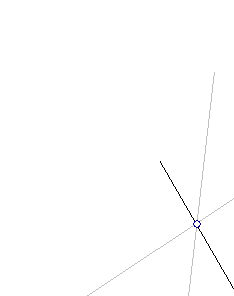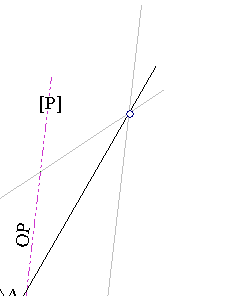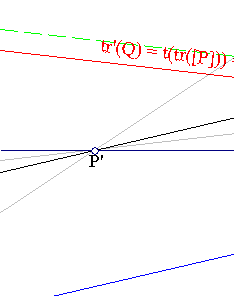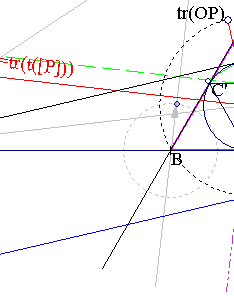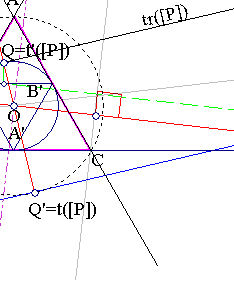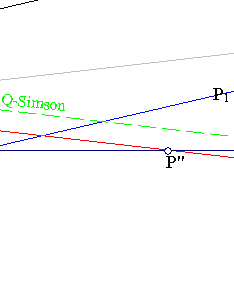The tangency of tr(P) to the incircle follows from section 8. The passing of tr'(Q) (resp. tr(Q')) through O follows from section 3. The identification of the contact point Q with the t'([P]) follows from IncircleTangents.html . From there also we have the parallelity of line tr'(Q) to the Simson line of Q with respect to triangle A'B'C'.
The relations between the elements of ABC and A'B'C' result from the projective invariance discussed in the previous section. The projectivity used is the anti-homothety F sending A'B'C' to ABC. The point at infinity [P] satisfies F([P])=[P], hence t([P])=t(F([P]))=F(t'([P]))=F(Q)=Q'.
The statement on OP is a consequence of the previous statements. Line OP is orthogonal to the Q'-Simson line with respect to ABC and its tripole is the point on the circumcircle of ABC such that the corresponding Simson line is orthogonal to it. According to well known property of Simson lines (see SimsonDiametral.html ) tr(OP) must be the diametral of t([P]).
The statement in  follows from the previous statements which relate the directions of the various lines. An easy angle chasing argument shows that t([P]) and t([P1]) are symmetric with respect to line tr'(Q).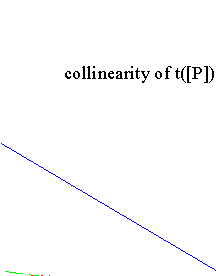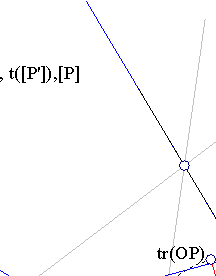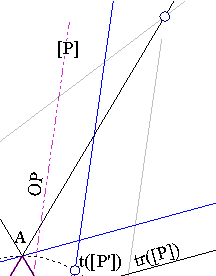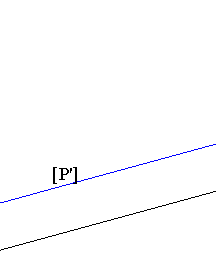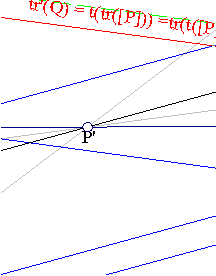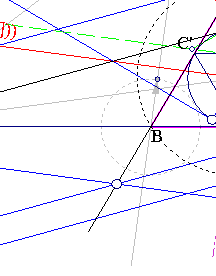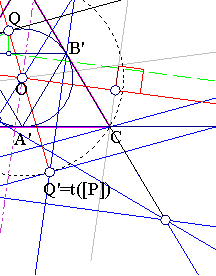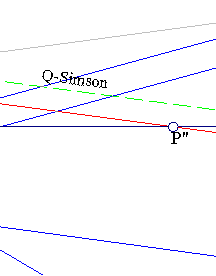##11. The general image

The general image results by mapping the system (A0B0C0, O) of an equilateral and its centroid to the system (ABC,K) of an arbitrary triangle and an arbitrary point K. The map used for this is the uniquely defined projectivity F mapping points {A0,B0,C0,O} correspondingly to {A,B,C,K}. The figure resulting by applying the map to the various elements of the above figure is commented in IsotomicGeneral.html .

##12. Remarks

Two particular cases of generalized isogonal transformations are well known and studied in detail:
i) The case in which K = I is the incenter. In this case the transformation is the standard isogonal conjugation.
ii) The case in which K = G is the centroid. In this case the transformation is the standard isotomic conjugation.

Note that these transformations are special quadratic Cremona transformations of the projective plane. The more general class of these transformations consists of birational transformations of the projective plane onto itself. Such transformations are described by homogeneous functions yi = fi(x1,x2,x3) of a certain degree r, the same for all three {f1, f2, f3}.
An example in which f1, f2 are quadratic but f3 is linear is studied in CircleBundleTransformation.html .

CircleBundleTransformation.html
IncircleTangents.html
InconicsTangents.html
IsotomicConicOfLine.html
IsotomicGeneral.html
ProjectiveCoordinates.html
SimsonDiametral.html
TriangleCircumconics.html
TriangleCircumconics2.html
TriangleConics.html
Trilinears.html